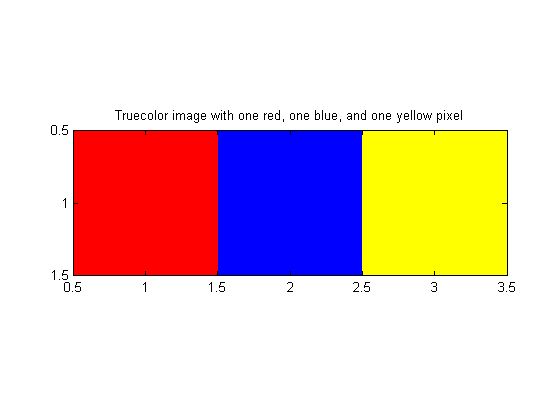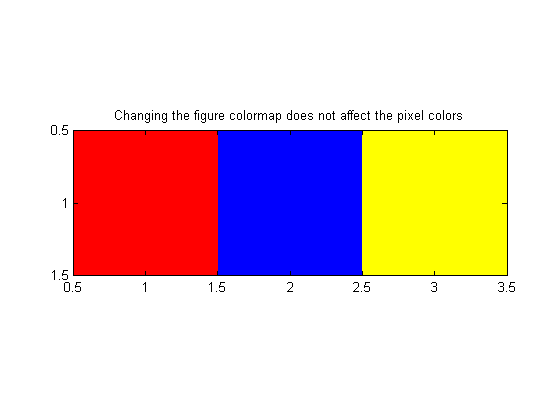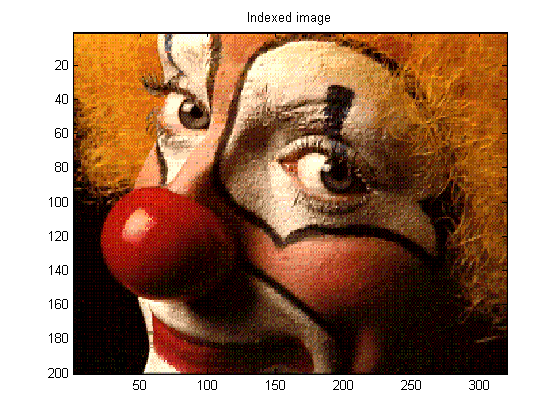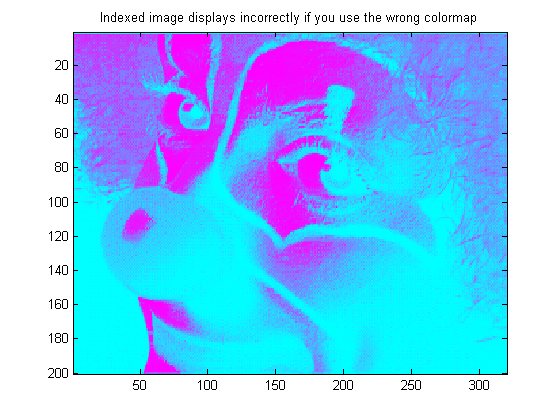# All about pixel colors: Truecolor and indexed images

Last week I posed this question: How does MATLAB associate the value of a particular matrix element with a color displayed on the screen? Let's start by exploring MATLAB's two basic pixel-color display models:

• Matrix element values specify pixel colors directly
• Matrix element values specify pixel colors indirectly, through the figure's colormap

## Truecolor images

The matrix (or array) of pixel values is stored in the Handle Graphics image object's CData property. If the CData array is a three-dimensional array with size M-by-N-by-3, then the pixel values specify the colors directly as a mix of red (first plane), green (second plane), and blue (third plane). We sometimes call such an image a "truecolor" image. (I believe this term originated in the computer graphics display industry. Someone please correct me if I'm wrong.)

Here's an illustrative image with just three pixels: red, blue, and yellow.

plane_1 = [1 0 1];
plane_2 = [0 0 1];
plane_3 = [0 1 0];
rgb = cat(3, plane_1, plane_2, plane_3);
size(rgb)

image(rgb)
axis image
title('Truecolor image with one red, one blue, and one yellow pixel')
ans =

1     3     3With truecolor images, changing the colormap has no effect on the image colors displayed.

colormap(hot)
title('Changing the figure colormap does not affect the pixel colors')## Indexed images

If the image CData is two-dimensional, then the CData values are treated as lookup indices into the figure's colormap. As an example, let's use an indexed image that ships with MATLAB, clown.mat (Ned's favorite).

s = load('clown')  % This the functional form of load.  This form returns
% a structure whose fields are the variables stored
% in the MAT-file.
s =

X: [200x320 double]
map: [81x3 double]
caption: [2x1 char]



The X and map variables stored in clown.mat are both necessary to display the image correctly. X contains the pixel values, and map contains the associated colormap.

To get the color of the (5,5) pixel, first see what X(5,5) is:

s.X(5,5)
ans =

61



Then use that value as a row index into the colormap matrix, map:

s.map(61,:)
ans =

0.9961    0.5781    0.1250



So the (5,5) pixel has a lot of red, some green, and a small amount of blue.

Displaying the image requires two MATLAB commands, one to create the image and one to set the figure's colormap:

image(s.X)
colormap(s.map)
title('Indexed image')Unlike truecolor images, indexed images are affected by changes in the figure's colormap.

colormap(cool)
title('Indexed image displays incorrectly if you use the wrong colormap')In my first post on this subject, I suggested that there might really be three pixel-color display models in MATLAB instead of two. The third display model is a variation of the indexed image model.

I'll talk about that next time.

Published with MATLAB® 7.1

|